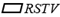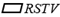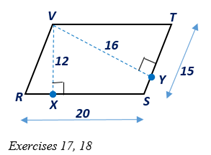Chapter 4.1, Problem 17E### Elementary Geometry for College St...

6th Edition
Daniel C. Alexander + 1 other
ISBN: 9781285195698

#### Solutions

Chapter
Section### Elementary Geometry for College St...

6th Edition
Daniel C. Alexander + 1 other
ISBN: 9781285195698
Textbook Problem
1 views

# In Exercises 17 and 18, considerwith V X - ⊥ R S - and V Y - ⊥ S T - .a) Which line segment is the altitude ofwith respect to base S T - ?b) Which number is the height ofwith respect to base S T - ?To determine

a.

To find:

The line segment which is the altitude of RSTV with respect to base ST¯.

Explanation

Given:

Consider RSTV with VX¯RS¯ and VY¯ST¯ and the diagram as shown below.

Definition:

An altitude of a parallelogram is any line segment drawn from one side so that it is perpendicular to the nonadjacent side or to an extension of that side.

In the RSTV, given that VX¯RS

To determine

b.

To find:

The height of RSTV with to base ST¯.

### Still sussing out bartleby?

Check out a sample textbook solution.

See a sample solution

#### The Solution to Your Study Problems

Bartleby provides explanations to thousands of textbook problems written by our experts, many with advanced degrees!

Get Started

#### In Exercises 49-62, find the indicated limit, if it exists. 54. limb3b+1b+3

Applied Calculus for the Managerial, Life, and Social Sciences: A Brief Approach

#### Use sets toshow that 06=0.

Mathematical Excursions (MindTap Course List)

#### What is the lowest score in the following distribution?

Essentials of Statistics for The Behavioral Sciences (MindTap Course List)

#### Prove the identity. 43. sin(2+x)=cosx

Single Variable Calculus: Early Transcendentals, Volume I

#### The unit vector in the direction of is:

Study Guide for Stewart's Multivariable Calculus, 8th

#### For y = f (u) and u = g(x), dydx=dydududx.

Study Guide for Stewart's Single Variable Calculus: Early Transcendentals, 8th